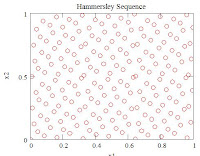## 4.05.2011

### Space Filling Design of Experiments

In previous issues of the TeraSigma Consulting Newsletter, The Power of Collaboration, we have described how to optimize the Design of Experiments while improving the exploration area, with the Second Order Design of Experiments. Whereas the objective of these designs is to cover the most area of experimentation with a minimum amount of trials, what happens when the amount of trials is not a restriction to perform our experimentation phase? for instance, when we can perform computer models and try them throughout the design space.

Then the challenge is to make sure the experimental trials are designed to cover the entire space without missing any region that may contain a special effect; the theory to define those experimental trials is summarized in the Space Filling Design of Experiments concepts.

Space filling designs are useful for modeling systems that are deterministic or near-deterministic. One example of a deterministic system is a computer simulation. A goal of designed experiments on these systems is to find a simpler empirical model that adequately predicts the behavior of the system over factor limited ranges

In experiments on deterministic systems, there is no variance but there is bias. Bias is the difference between the approximation model and the true mathematical function. Space filling designs attempt is to bound the bias two possible ways:

• Spread the design points as far from each other as possible consistent with staying inside the experimental boundaries.
• Space the points evenly over the region of interest.

One of the most common methods to design Space Filling DOE is the Latin Hypercube. It is a particular Monte Carlo Sampling; more precisely it is a constrained Monte Carlo sampling scheme. While pure Monte Carlo Simulation relies on random number generation to define the level of a given factor, Latin Hypercube is constrained to get that level from a uniform statistical distribution. This method maximizes the minimum distance between design points but requires even spacing of the levels of each factor. Other distributions can be chosen for particular factors, creating special Latin Hypercube designs; nevertheless, the uniform distribution is the most common approach, especially in the design phase of the experimentation, when the distribution across the range of a factor is still unknown.Latin Hypercube (n=200)Monte Carlo (n=200)

How to create a Latin Hypercube design? A Latin Hypercube is easiest to visualize in 2 dimensions - extends to N-dimensions.

Based on a Latin Square, divide each axis of the design space into n equally sized (equal probability) cells, e.g. let n=3 and 2 Xs, each with range [0, 1]

1.       Permute the values 1, … n, to fill the columns
2.       Randomly choose an integer 1-n
3.       Randomly place a point in each of those cells
·         Degree of “space filling” depends on integer chosen and placement of point in each cell
·         Some choices can result in correlations among the Xs
·         Modifications exist to get better control over space filling property and correlation of Xs

How many points on a Latin Hypercube design are recommended? Whereas in the second order DOE the number of trials grow exponentially 2N, where N is the number of factors, the recommendation for Latin Hypercube designs is 10(N).

It is also recommended to analyze the results from a Latin Hypercube design in a sequenced sampling order; this allows the analyst to verify convergence of data and to identify cofounding and bias in an early manner. The points should gradually fill the space design.

There are many commercial statistical software packages that can handle Space Filling Design of Experiments, such as JMP and Minitab. Should you have any question regarding Latin Hypercube or any other Space Filling DOE, don’t hesitate to contact the consultants at TeraSigma Consulting, The Power of Collaboration.